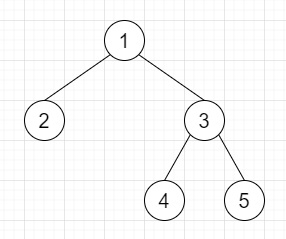# Serialize and Deserialize Binary Tree in C++

Suppose we have one binary tree and we have to serialize and deserialize them. As we know that the serialization is the process of converting a data structure or object into a sequence of bits so we can store them in a file or memory buffer, and that can be reconstructed later in the same or another computer environment.

Here we have to devise an algorithm to serialize and deserialize binary tree. The binary tree is a rooted tree in which each node has no more than 2 children.

So, if the input is likethen the output will be Serialize − 1 2 3 4 5 N N N N N N Deserialized Tree: 4 2 5 1 3.

To solve this, we will follow these steps −

• Define a function serialize(), this will take root,

• ret := blank string

• Define one queue q

• insert root into q

• while (not q is empty), do −

• curr = first element of q

• delete element from q

• if curr is not available, then −

• ret := ret concatenate "N"

• ret := ret concatenate blank space

• ret := ret + value of curr

• ret := ret + blank space

• left of curr at the end of q

• right of curr at the end of q

• return ret

• Define a function deserialize(), this will take data,

• if data is same as 'N', then −

• return NULL

• temp := empty string

• Define an array v

• for initialize i := 0, when i − size of data, update (increase i by 1), do −

• if data[i] is same as blank space, then −

• insert temp at the end of v

• temp := blank string

• temp := temp + data[i]

• newRoot = new node with v

• Define one queue q

• insert newRoot into q

• i := 1

• while (not q is empty and i < size of v), do −

• parent = first element of q

• delete element from q

• if v[i] is not equal to "N", then −

• left of parent := new node with v[i]

• insert left of parent into q

• (increase i by 1)

• if v[i] is not equal to "N", then −

• right of parent := new node with v[i]

• insert right of parent into q

• (increase i by 1)

• return newRoot

## Example

Let us see the following implementation to get a better understanding −

Live Demo

#include <bits/stdc++.h>
using namespace std;
class TreeNode {
public:
int val;
TreeNode *left, *right;
TreeNode(int data) {
val = data;
left = NULL;
right = NULL;
}
};
void insert(TreeNode **root, int val) {
queue<TreeNode *> q;
q.push(*root);
while (q.size()) {
TreeNode *temp = q.front();
q.pop();
if (!temp->left) {
if (val != NULL)
temp->left = new TreeNode(val);
else
temp->left = new TreeNode(0);
return;
}
else {
q.push(temp->left);
}
if (!temp->right) {
if (val != NULL)
temp->right = new TreeNode(val);
else
temp->right = new TreeNode(0);
return;
}
else {
q.push(temp->right);
}
}
}
TreeNode *make_tree(vector<int> v) {
TreeNode *root = new TreeNode(v);
for (int i = 1; i < v.size(); i++) {
insert(&root, v[i]);
}
return root;
}
void inord(TreeNode *root) {
if (root != NULL) {
inord(root->left);
cout << root->val << " ";
inord(root->right);
}
}
class Codec {
public:
string serialize(TreeNode *root) {
string ret = "";
queue<TreeNode *> q;
q.push(root);
while (!q.empty()) {
TreeNode *curr = q.front();
q.pop();
if (!curr) {
ret += "N";
ret += " ";
continue;
}
ret += to_string(curr->val);
ret += " ";
q.push(curr->left);
q.push(curr->right);
}
return ret;
}
TreeNode *deserialize(string data) {
if (data == 'N')
return NULL;
string temp = "";
vector<string> v;
for (int i = 0; i < data.size(); i++) {
if (data[i] == ' ') {
v.push_back(temp);
temp = "";
continue;
}
temp += data[i];
}
TreeNode *newRoot = new TreeNode(stoi(v));
queue<TreeNode *> q;
q.push(newRoot);
int i = 1;
while (!q.empty() && i < v.size()) {
TreeNode *parent = q.front();
q.pop();
if (v[i] != "N") {
parent->left = new TreeNode(stoi(v[i]));
q.push(parent->left);
}
i++;
if (v[i] != "N") {
parent->right = new TreeNode(stoi(v[i]));
q.push(parent->right);
}
i++;
}
return newRoot;
}
};
main() {
Codec ob;
vector<int> v = {1,2,3,4,5};
TreeNode *root = make_tree(v);
cout << "Given Tree: ";
inord(root);
cout << endl;
string ser = ob.serialize(root);
cout << "Serialize: " << ser << endl;
TreeNode *deser = ob.deserialize(ser);
cout << "Deserialized Tree: ";
inord(root);
}

## Input

1,2,3,4,5

## Output

Given Tree: 4 2 5 1 3
Serialize: 1 2 3 4 5 N N N N N N
Deserialized Tree: 4 2 5 1 3